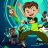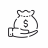# Auto MTF for studies#### subharmonic

##### New member
VIP
This can be adapted to any study but I generally use 5/30 charts intraday (find main move on 30 and look for entries on the small time frame) and day/week. This will automatically set the anchor indicator to the higher
Code:
``````declare lower;
def AP = GetAggregationPeriod();
def AP1 = if AP >= AggregationPeriod.thirty_min then AggregationPeriod.Week else  AggregationPeriod.thirty_min;
input over_bought = 40.0;
input over_sold = -40.0;
input percentDLength = 3;
input percentKLength = 5;

def min_low = lowest(low(period = AP1), percentKLength);
def max_high = highest(high(period = AP1), percentKLength);
def rel_diff = close(period = AP1) - (max_high + min_low)/2;
def diff = max_high - min_low;

def avgrel = expaverage(expaverage(rel_diff, percentDLength), percentDLength);
def avgdiff = expaverage(expaverage(diff, percentDLength), percentDLength);

plot SMI = if avgdiff != 0 then avgrel / (avgdiff / 2) * 100 else 0;
smi.setDefaultColor(getColor(1));

plot AvgSMI = expaverage(smi, percentDLength);
avgsmi.setDefaultColor(getcolor(5));

plot overbought = over_bought;
overbought.setDefaultColor(getcolor(5));

plot oversold = over_sold;
oversold.setDefaultColor(getcolor(5));``````

or for you TMO users
Code:
``````# TMO ((T)rue (M)omentum (O)scilator)
# Mobius
# V01.05.2018
#hint: TMO calculates momentum using the delta of price. Giving a much better picture of trend, tend reversals and divergence than momentum oscillators using price.

declare lower;

input length = 14;
input calcLength = 5;
input smoothLength = 3;
def AP = GetAggregationPeriod();

def MTF = if AP >= AggregationPeriod.thirty_min then AggregationPeriod.Week else  AggregationPeriod.thirty_min;

def o = open(period = MTF);
def c = close(period = MTF);
def data = fold i = 0 to length
with s
do s + (if c > GetValue(o, i)
then 1
else if c < GetValue(o, i)
then - 1
else 0);
def EMA5 = ExpAverage(data, calcLength);
plot Main = ExpAverage(EMA5, smoothLength);
plot Signal = ExpAverage(Main, smoothLength);
Main.AssignValueColor(if Main > Signal
then Color.Green
else Color.RED);
Signal.AssignValueColor(if Main > Signal
then Color.Green
else Color.RED);
Signal.HideBubble();
Signal.HideTitle();

plot zero = if isNaN(c) then double.nan else 0;
zero.SetDefaultColor(Color.gray);
zero.hideBubble();
zero.hideTitle();
plot ob = if isNaN(c) then double.nan else round(length * .7);
ob.SetDefaultColor(Color.gray);
ob.HideBubble();
ob.HideTitle();
plot os = if isNaN(c) then double.nan else -round(length * .7);
os.SetDefaultColor(Color.gray);
os.HideBubble();
os.HideTitle();

Would also be good to keep a 200 daily SMA on all your charts except higher times than a daily.

•MerryDayAuto Volatility Standard Deviation Levels for ThinkorSwim Custom 19Scan / Watchlist auto-refresh possible? Questions 7